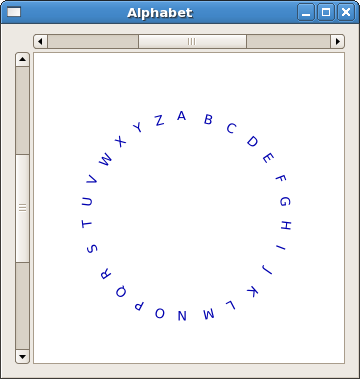## 6.3 The Layout Container

Until now packing of widgets has been done either through sequencing in horizontal or vertical boxes, or in a table. You can, however, also place widgets in any position you like, using a `Fixed` or a `Layout` widget. Use of the `Fixed` container is not recommended, because it does not resize well.

The Layout container is similar to the Fixed container except that it implements an infinite (where infinity is less than 2^32) scrolling area. The X window system has a limitation where windows can be at most 32767 pixels wide or tall. The Layout container gets around this by doing some exotic stuff using window and bit gravities, so that you can have smooth scrolling even when you have many child widgets in your scrolling area. Since it is used in a scrolled window, the disadvantages of the `Fixed` widget do not apply.

A Layout container is created using:

```layoutNew :: Maybe Adjustment -> Maybe Adjustment -> IO Layout
```

As you can see, you can optionally specify the Adjustment objects that the Layout widget will use for its scrolling.

You can add and move widgets in the Layout container using the following two functions:

```layoutPut :: (LayoutClass self, WidgetClass childWidget)
=> self -> childWidget -> Int -> Int -> IO ()
layoutMove :: (LayoutClass self, WidgetClass childWidget)
=> self -> childWidget -> Int -> Int -> IO ()
```

The first argument is the x position, the second the y position. The top left is (0,0), x grows from left to right, and y from top to bottom.

The size of the `Layout` container can be set using the next function:

```layoutSetSize :: LayoutClass self => self  -> Int -> Int -> IO ()
```

The first argument is the width of the entire scrollable area, the second the height.

In the example we place a list of labels, each with an upper case letter of the alphabet, in a circle around a chosen centre. The labels are positioned perpendicular to the radius using the Gtk2Hs function:

```labelSetAngle :: labelClass self => self -> Double -> IO ()
```

The angle is in degrees, measured counterclockwise.The layout widget is placed into a scrolled window with `containerAdd` because it is scrollable, and so does not need a view port, as in Chapter 6.1. The labels are positioned using angular coordinates, which are then transformed into Cartesian coordinates with the Prelude `sin` and `cos` functions. These take radians as their arguments (between 0 and (2 * pi)). Width and Height in the example are parametrized, as is the list to be displayed. Furthermore, in `main` the corners of the `Layout` are marked, so you can easily experiment with its size, if you want. Note that the actual marker has been replaced with '+' here, because the validator complained.

```import Graphics.UI.Gtk

main :: IO ()
main = do
initGUI
window <- windowNew
set window [windowTitle := "Alphabet" , windowDefaultWidth := 350,
windowDefaultHeight := 350 , containerBorderWidth := 10]
sw <- scrolledWindowNew Nothing Nothing
set sw [scrolledWindowPlacement := CornerBottomRight,
scrolledWindowHscrollbarPolicy := PolicyAutomatic,
scrolledWindowVscrollbarPolicy := PolicyAutomatic ]

layt <- layoutNew Nothing Nothing
layoutSetSize layt myLayoutWidth myLayoutHeight
widgetModifyBg layt StateNormal (Color 65535 65535 65535)

upleft  <- labelNew (Just "+(0,0)")
layoutPut layt upleft 0 0
upright <- labelNew (Just ("+(" ++ (show (myLayoutWidth - 50)) ++",0)"))
layoutPut layt upright (myLayoutWidth -50)  0
dwnright <- labelNew (Just ("+(0," ++ (show (myLayoutHeight -20)) ++ ")"))
layoutPut layt dwnright 0 (myLayoutHeight -20)
dwnleft <- labelNew (Just ("+(" ++ (show(myLayoutWidth -70)) ++ "," ++
(show (myLayoutHeight -20)) ++ ")"))
layoutPut layt dwnleft (myLayoutWidth -70) (myLayoutHeight - 20)

labels <- sequence \$ map (labelNew . Just) txtls
sequence_ \$ map (\x -> widgetModifyFg x StateNormal (Color 0 0 45000)) labels

let wnums = zip labels [0..]
sequence_ \$ map (myLayoutPut layt) wnums

widgetShowAll window
onDestroy window mainQuit
mainGUI

-- parameters
myLayoutWidth :: Int
myLayoutWidth = 800

myLayoutHeight :: Int
myLayoutHeight = 800

txtls :: [String]
txtls = map (\x -> x:[]) ['A'..'Z']
-- end parameters

step :: Double
step = (2 * pi)/(fromIntegral (length txtls))

ox :: Int
ox =  myLayoutWidth `div` 2

oy :: Int
oy = myLayoutHeight `div` 2

radius = 0.25 * (fromIntegral ox)

angle :: Int -> Double
angle num = 1.5 * pi + (fromIntegral num) * step

num2x :: Int -> Int
num2x n = ox + relx where
relx = round \$ radius * (cos (angle n))

num2y :: Int -> Int
num2y n = oy + rely where
rely = round \$ radius * (sin (angle n))

myLayoutPut :: Layout -> (Label, Int) -> IO ()
myLayoutPut lt (lb, n) = do
layoutPut lt lb (num2x n) (num2y n)
labelSetAngle lb (letterAngle n)

letterAngle :: Int -> Double
letterAngle n = (270 - degree) where
degree = (angle n) * (180.0 /pi)
```